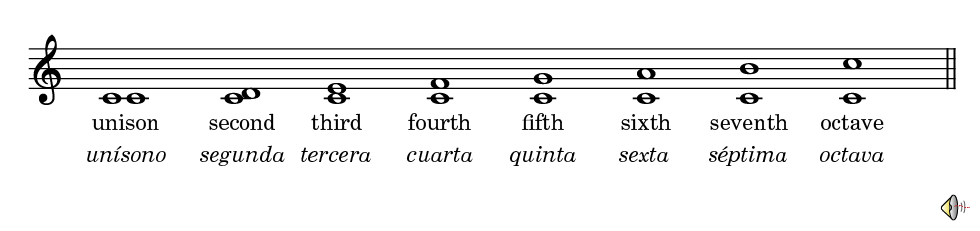By counting the number of notes in an interval we obtain its numerical size. The first and last notes must be counted. For example, from C to E we have a third (C-1, D-2, E-3). In the next figure you can see the relationship between the number of notes and the numerical size of intervals:However, not all intervals of the same numerical classification have the same size. Therefore, we need to specify the quality by finding the exact number of whole and half steps in the interval.

See Intervals in the Tutorial section.